# Selina Solutions Concise Mathematics Class 6 Chapter 23 Fundamental Concepts

Selina Solutions Concise Mathematics Class 6 Chapter 23 Fundamental Concepts help students get a clear idea of operations and methods to be followed in solving exercise problems, without any obstacles. The solutions explain basic concepts in an understandable language. Students, who want to acquire better conceptual knowledge, can follow shortcut tricks and important formulas covered in a stepwise manner. This also helps them to improve problem-solving abilities within a short duration. Selina Solutions Concise Mathematics Class 6 Chapter 23 Fundamental Concepts PDF can be downloaded, from the links provided here.

Chapter 23 explains the elementary treatment and operations on various terms in geometry, according to the latest ICSE syllabus. The main purpose of preparing solutions is to help students come out with better academic performance.

## Selina Solutions Concise Mathematics Class 6 Chapter 23 Fundamental Concepts Download PDF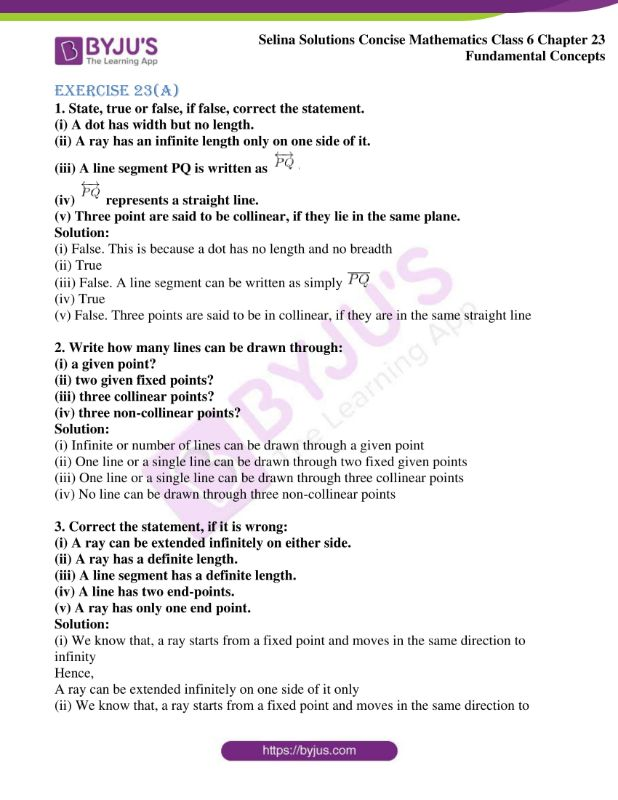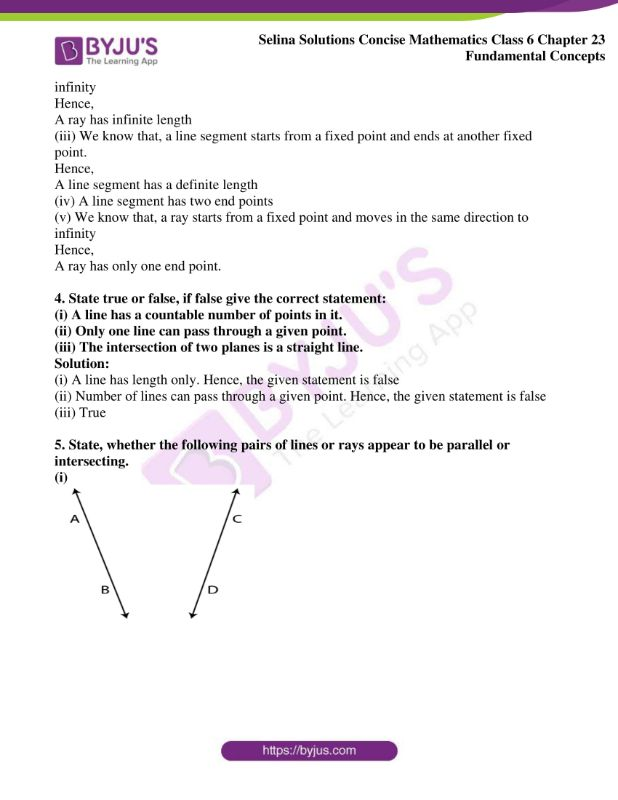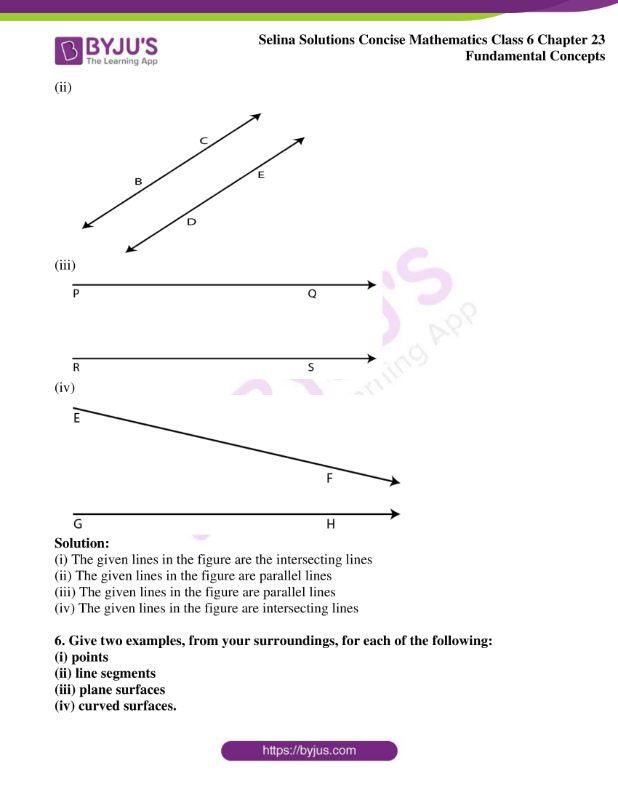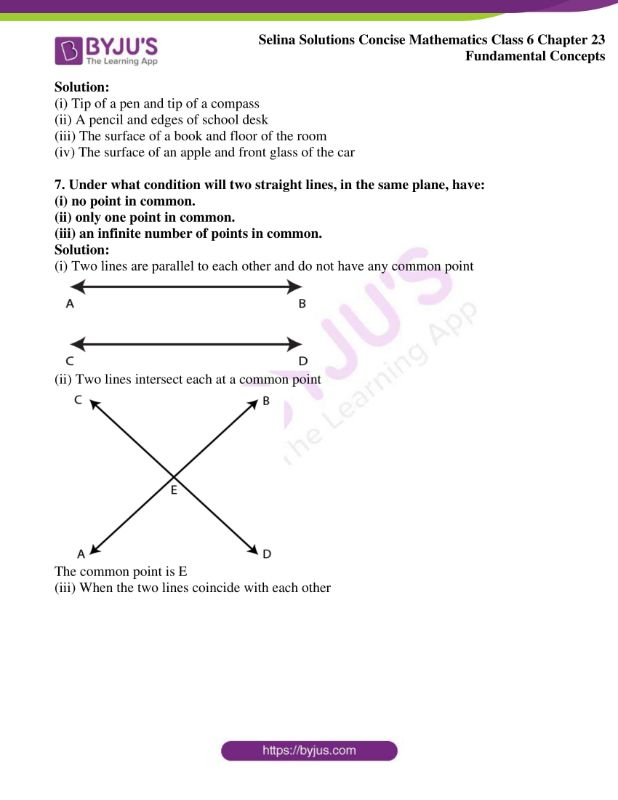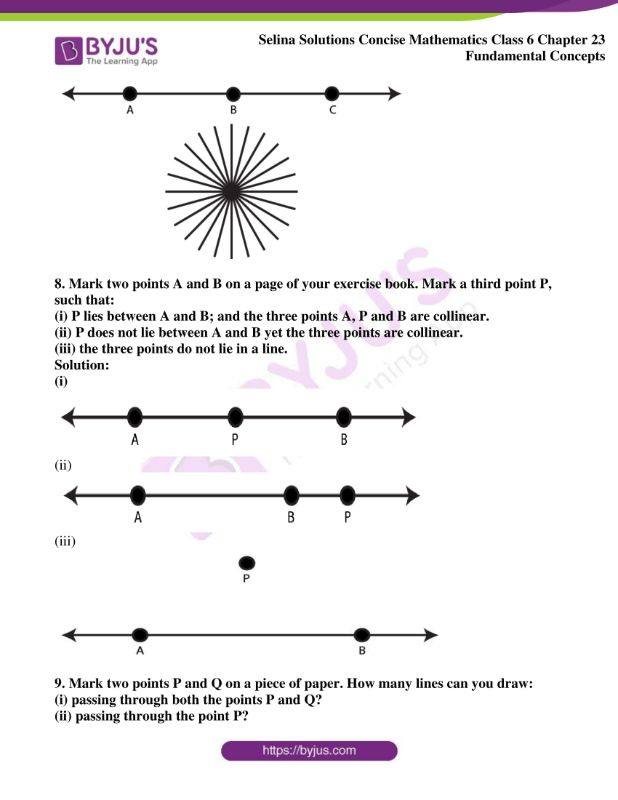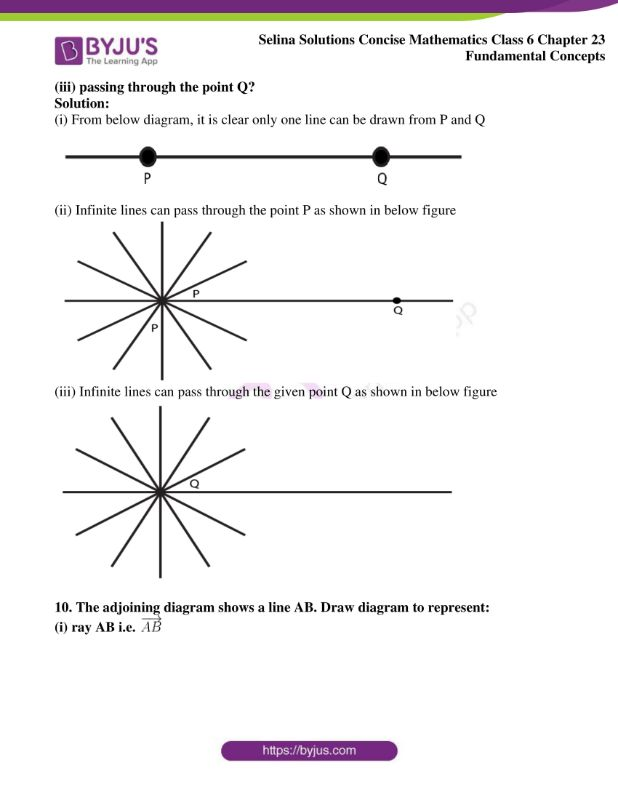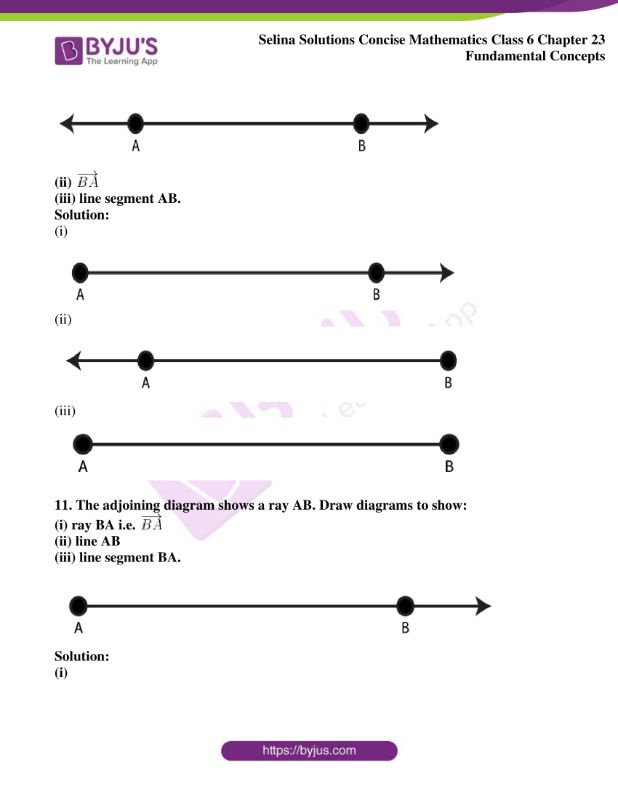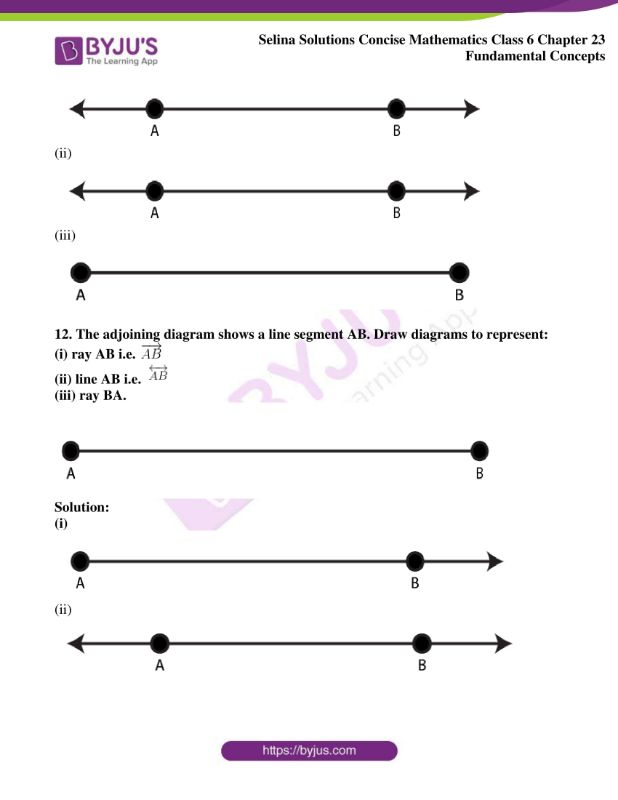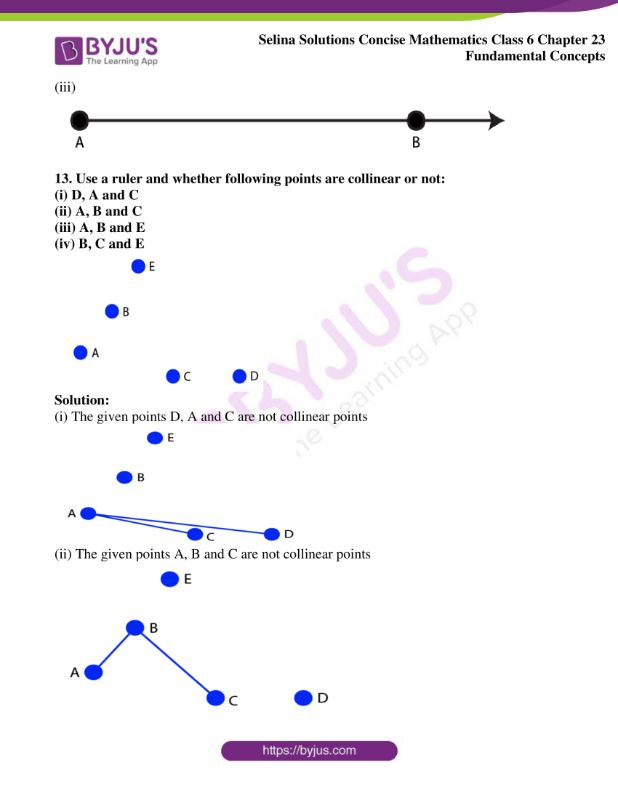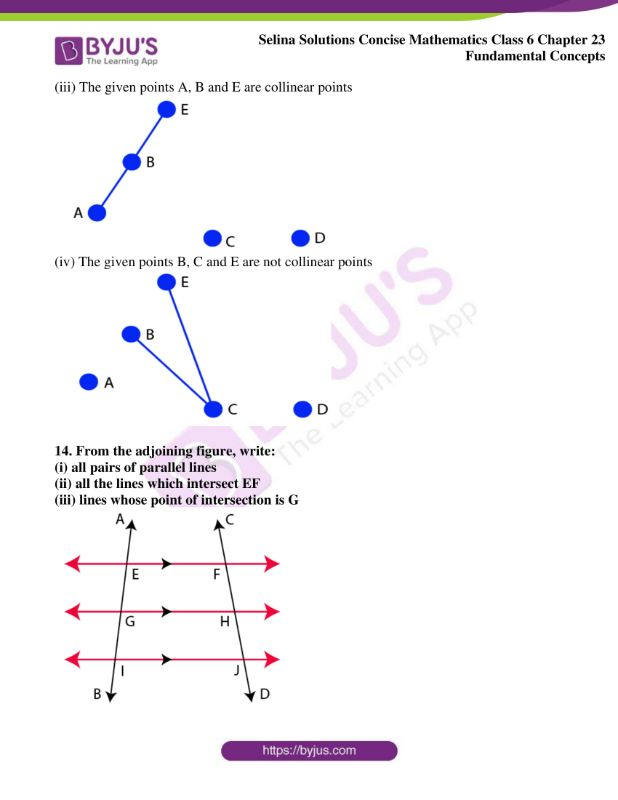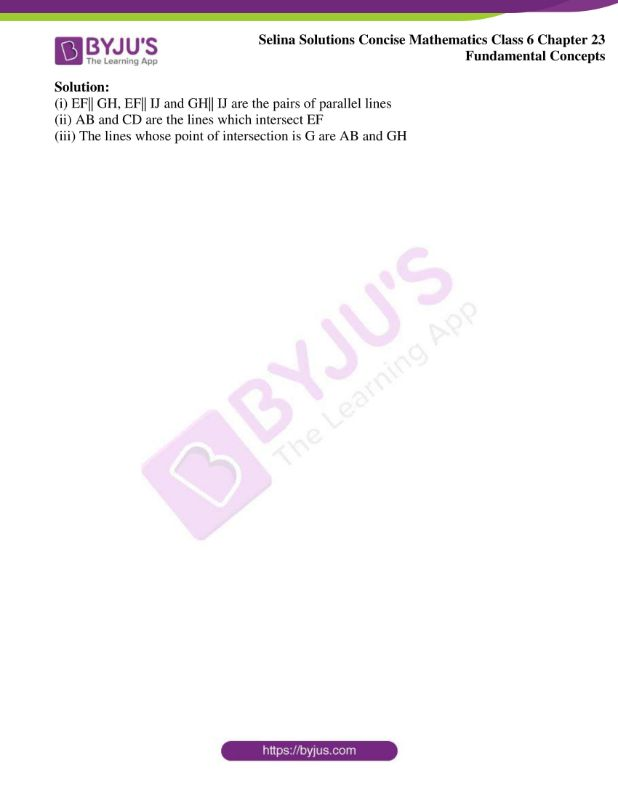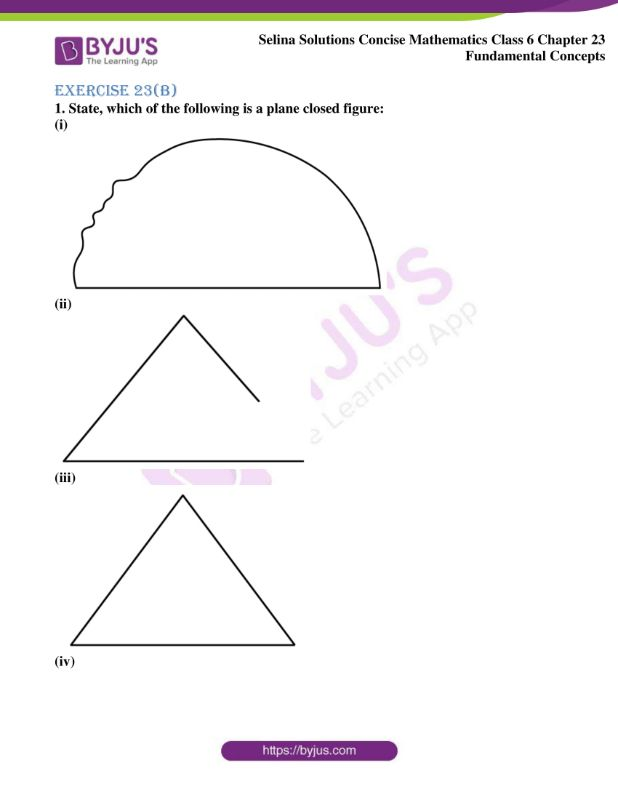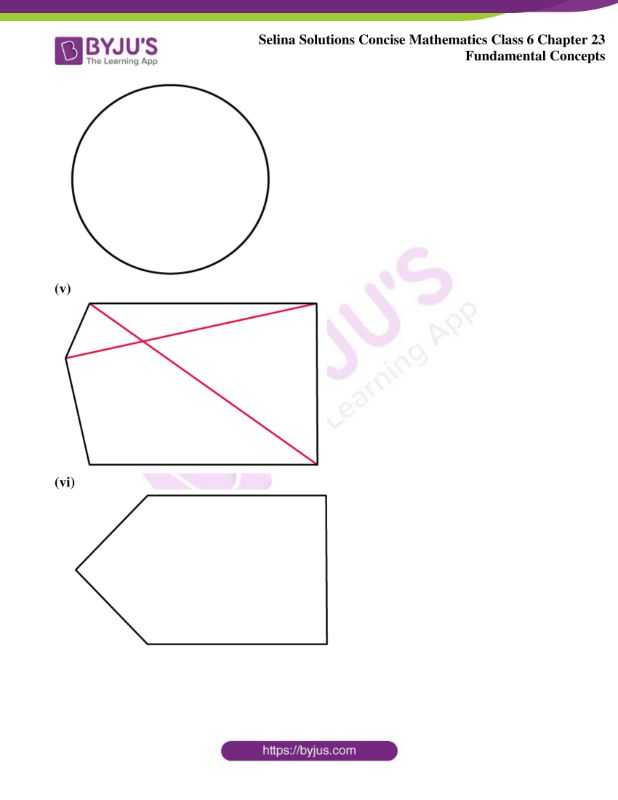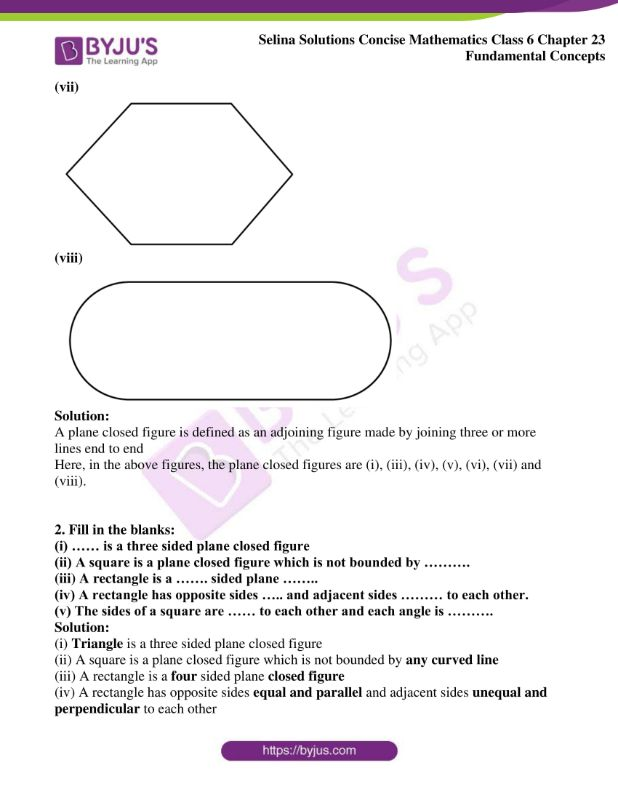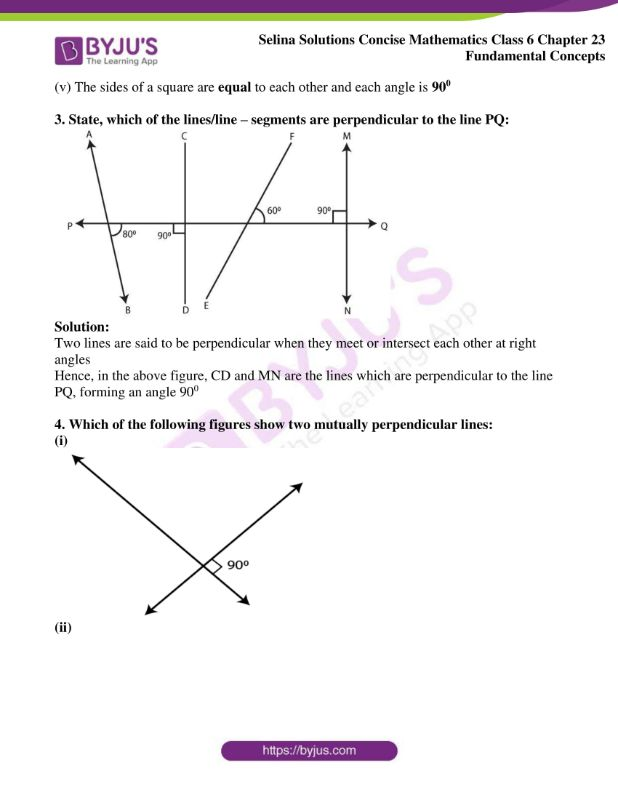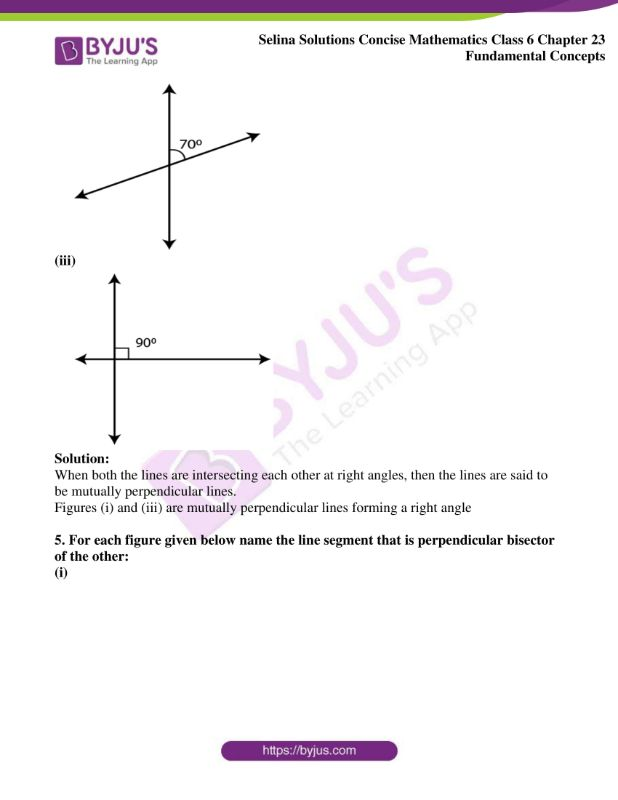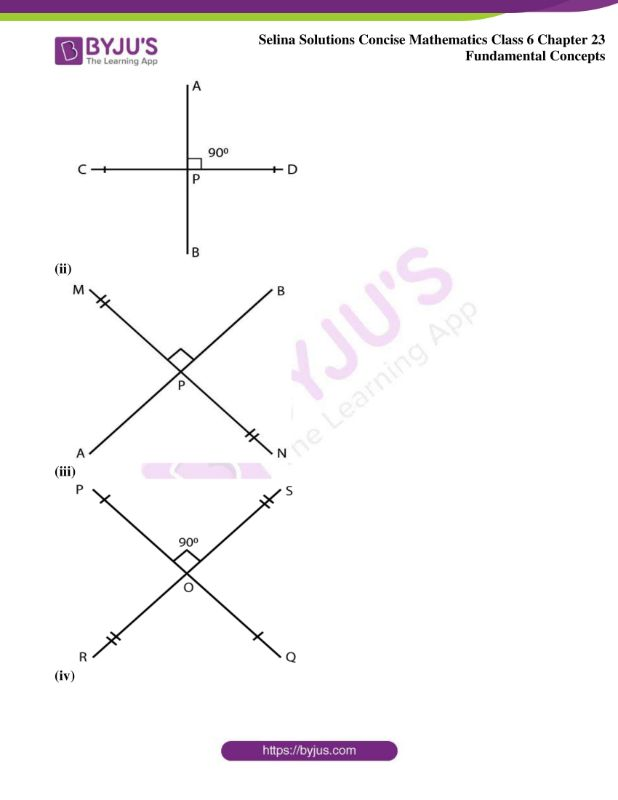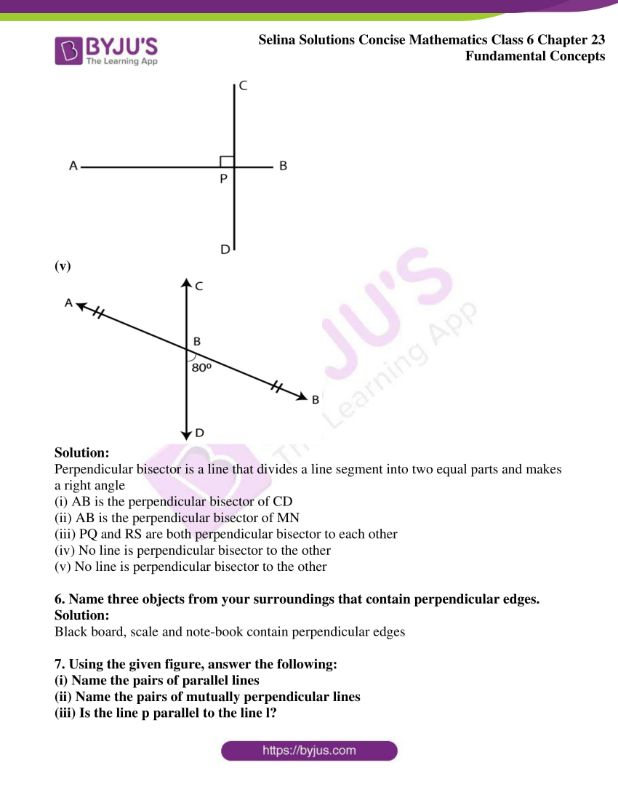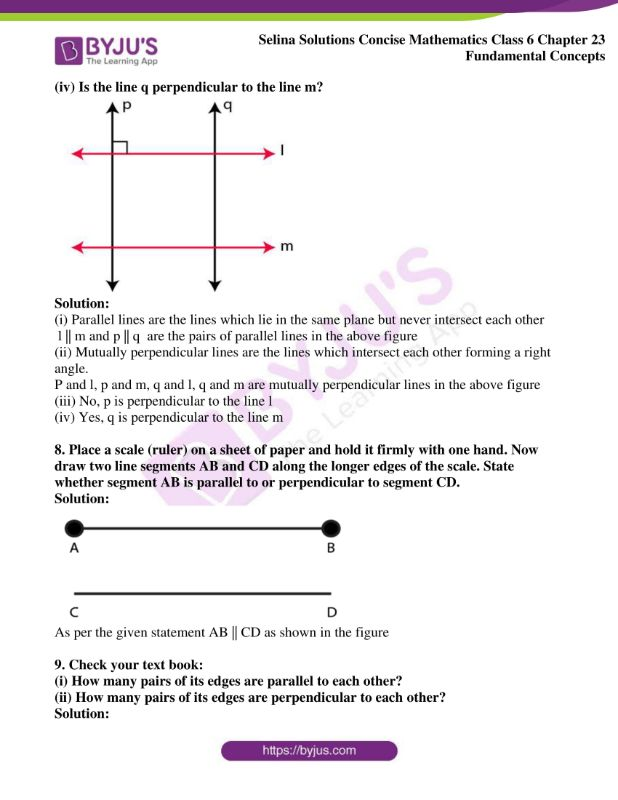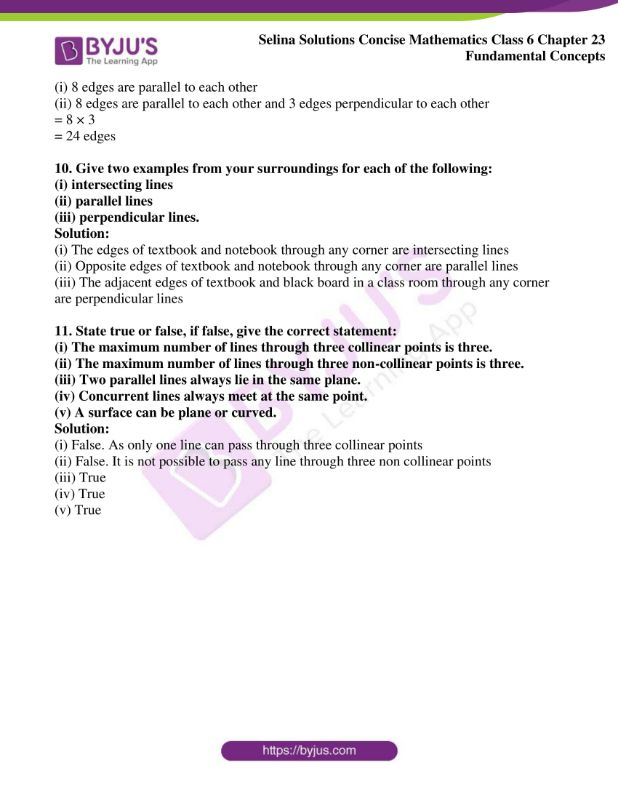### Exercises of Selina Solutions Concise Mathematics Class 6 Chapter 23 Fundamental Concepts

Exercise 23(A) Solutions

Exercise 23(B) Solutions

## Access Selina Solutions Concise Mathematics Class 6 Chapter 23 Fundamental Concepts

Exercise 23(A)

1. State, true or false, if false, correct the statement.

(i) A dot has width but no length.

(ii) A ray has an infinite length only on one side of it.

(iii) A line segment PQ is written as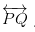(iv)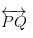represents a straight line.

(v) Three point are said to be collinear, if they lie in the same plane.

Solution:

(i) False. This is because a dot has no length and no breadth

(ii) True

(iii) False. A line segment can be written as simply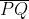(iv) True

(v) False. Three points are said to be in collinear, if they are in the same straight line

2. Write how many lines can be drawn through:

(i) a given point?

(ii) two given fixed points?

(iii) three collinear points?

(iv) three non-collinear points?

Solution:

(i) Infinite or number of lines can be drawn through a given point

(ii) One line or a single line can be drawn through two fixed given points

(iii) One line or a single line can be drawn through three collinear points

(iv) No line can be drawn through three non-collinear points

3. Correct the statement, if it is wrong:

(i) A ray can be extended infinitely on either side.

(ii) A ray has a definite length.

(iii) A line segment has a definite length.

(iv) A line has two end-points.

(v) A ray has only one end point.

Solution:

(i) We know that, a ray starts from a fixed point and moves in the same direction to infinity

Hence,

A ray can be extended infinitely on one side of it only

(ii) We know that, a ray starts from a fixed point and moves in the same direction to infinity

Hence,

A ray has infinite length

(iii) We know that, a line segment starts from a fixed point and ends at another fixed point.

Hence,

A line segment has a definite length

(iv) A line segment has two end points

(v) We know that, a ray starts from a fixed point and moves in the same direction to infinity

Hence,

A ray has only one end point.

4. State true or false, if false give the correct statement:

(i) A line has a countable number of points in it.

(ii) Only one line can pass through a given point.

(iii) The intersection of two planes is a straight line.

Solution:

(i) A line has length only. Hence, the given statement is false

(ii) Number of lines can pass through a given point. Hence, the given statement is false

(iii) True

5. State, whether the following pairs of lines or rays appear to be parallel or intersecting.

(i)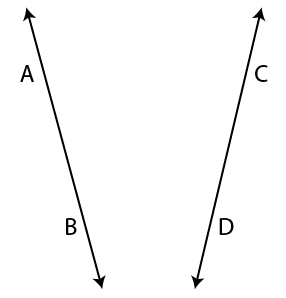(ii)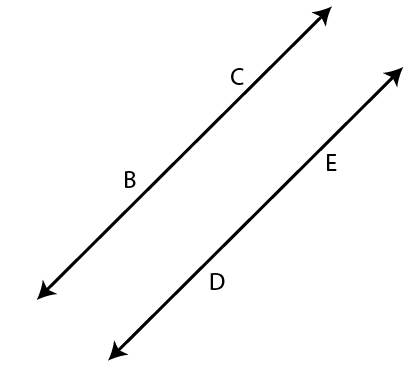(iii)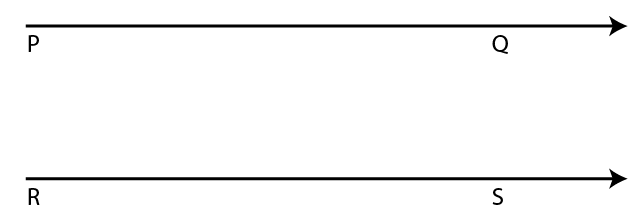(iv)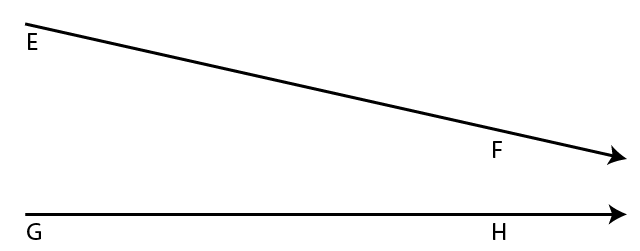Solution:

(i) The given lines in the figure are the intersecting lines

(ii) The given lines in the figure are parallel lines

(iii) The given lines in the figure are parallel lines

(iv) The given lines in the figure are intersecting lines

6. Give two examples, from your surroundings, for each of the following:

(i) points

(ii) line segments

(iii) plane surfaces

(iv) curved surfaces.

Solution:

(i) Tip of a pen and tip of a compass

(ii) A pencil and edges of school desk

(iii) The surface of a book and floor of the room

(iv) The surface of an apple and front glass of the car

7. Under what condition will two straight lines, in the same plane, have:

(i) no point in common.

(ii) only one point in common.

(iii) an infinite number of points in common.

Solution:

(i) Two lines are parallel to each other and do not have any common point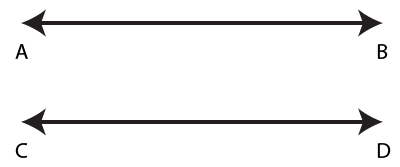(ii) Two lines intersect each at a common point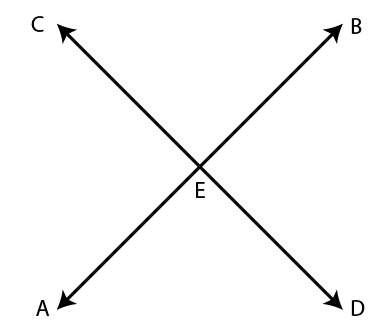The common point is E

(iii) When the two lines coincide with each other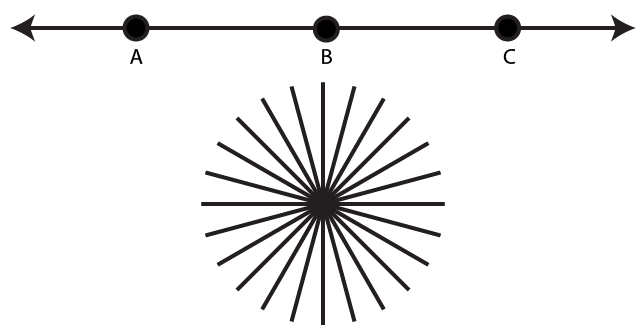8. Mark two points A and B on a page of your exercise book. Mark a third point P, such that:

(i) P lies between A and B; and the three points A, P and B are collinear.

(ii) P does not lie between A and B yet the three points are collinear.

(iii) the three points do not lie in a line.

Solution:

(i)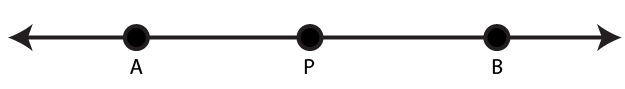(ii)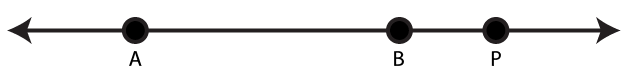(iii)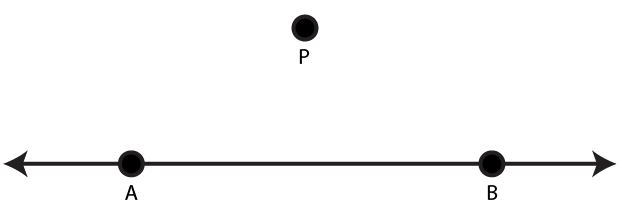9. Mark two points P and Q on a piece of paper. How many lines can you draw:

(i) passing through both the points P and Q?

(ii) passing through the point P?

(iii) passing through the point Q?

Solution:

(i) From below diagram, it is clear only one line can be drawn from P and Q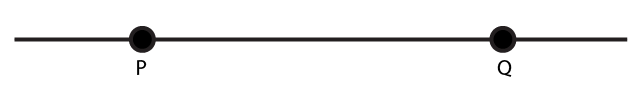(ii) Infinite lines can pass through the point P as shown in below figure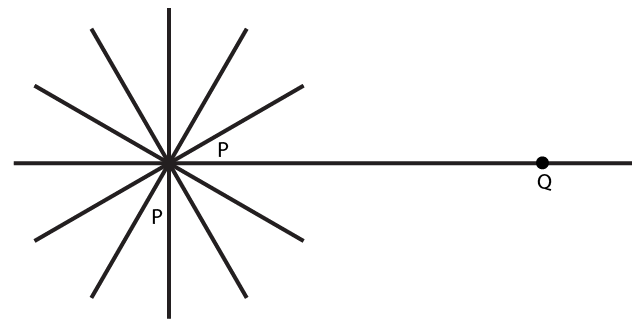(iii) Infinite lines can pass through the given point Q as shown in below figure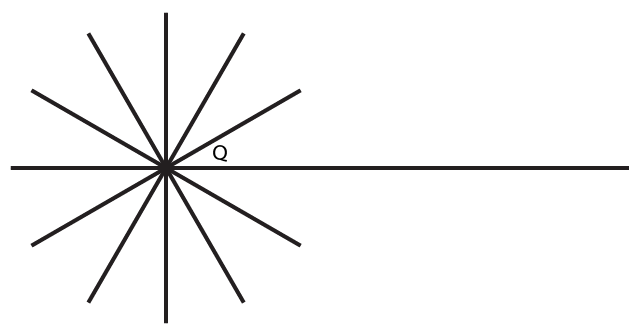10. The adjoining diagram shows a line AB. Draw diagram to represent:

(i) ray AB i.e.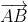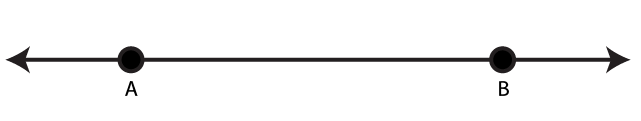(ii)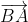(iii) line segment AB.

Solution:

(i)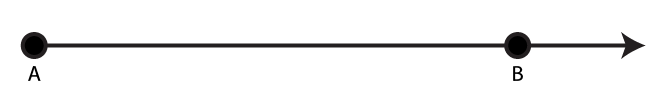(ii)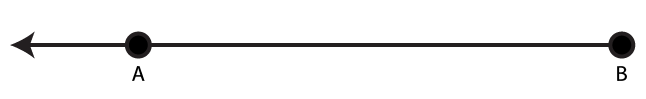(iii)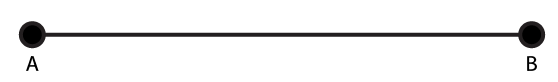11. The adjoining diagram shows a ray AB. Draw diagrams to show:

(i) ray BA i.e.(ii) line AB

(iii) line segment BA.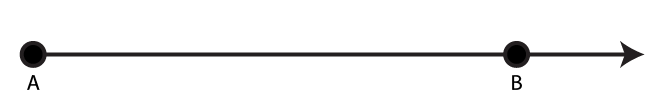Solution:

(i)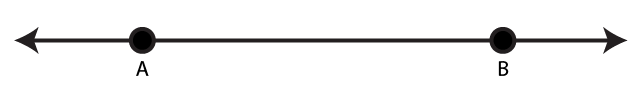(ii)(iii)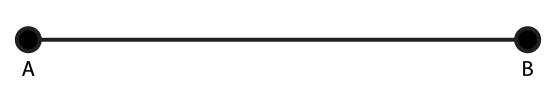12. The adjoining diagram shows a line segment AB. Draw diagrams to represent:

(i) ray AB i.e.(ii) line AB i.e.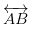(iii) ray BA.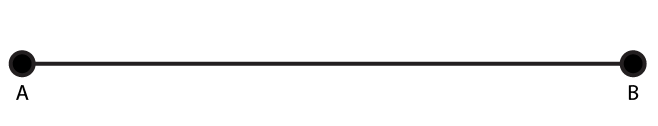Solution:

(i)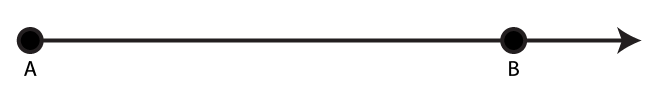(ii)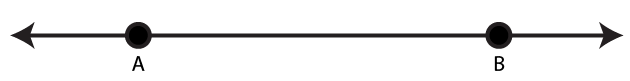(iii)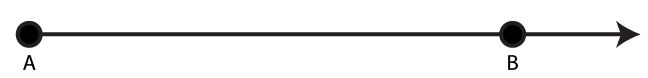13. Use a ruler and whether following points are collinear or not:

(i) D, A and C

(ii) A, B and C

(iii) A, B and E

(iv) B, C and E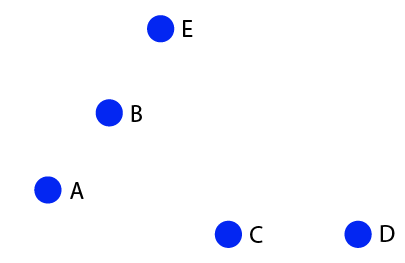Solution:

(i) The given points D, A and C are not collinear points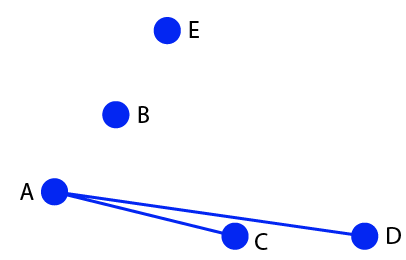(ii) The given points A, B and C are not collinear points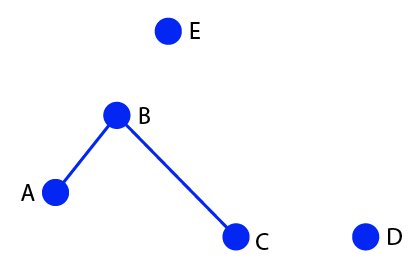(iii) The given points A, B and E are collinear points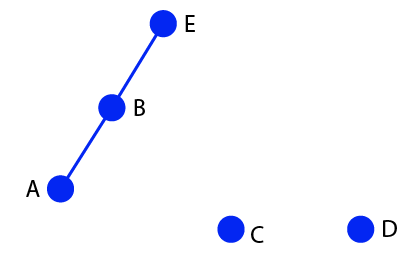(iv) The given points B, C and E are not collinear points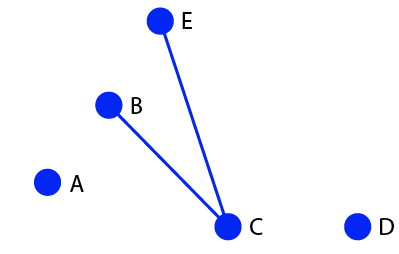14. From the adjoining figure, write:

(i) all pairs of parallel lines

(ii) all the lines which intersect EF

(iii) lines whose point of intersection is G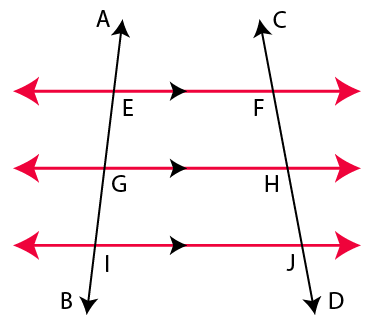Solution:

(i) EF|| GH, EF|| IJ and GH|| IJ are the pairs of parallel lines

(ii) AB and CD are the lines which intersect EF

(iii) The lines whose point of intersection is G are AB and GH

Exercise 23(B)

1. State, which of the following is a plane closed figure:

(i)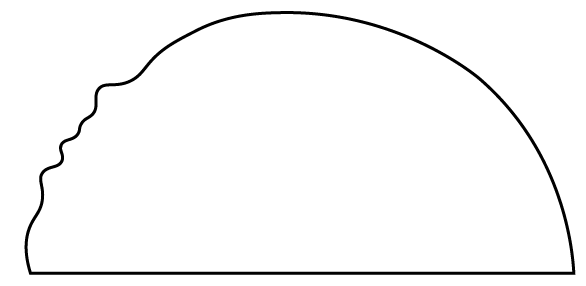(ii)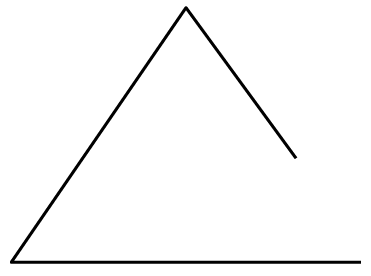(iii)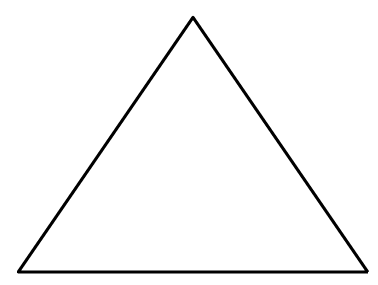(iv)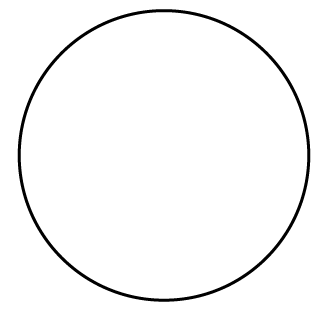(v)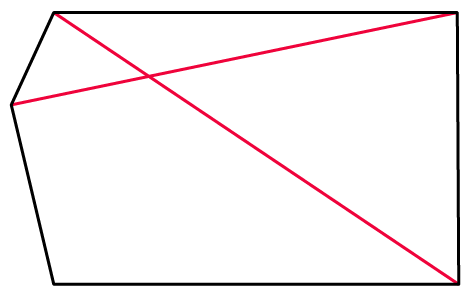(vi)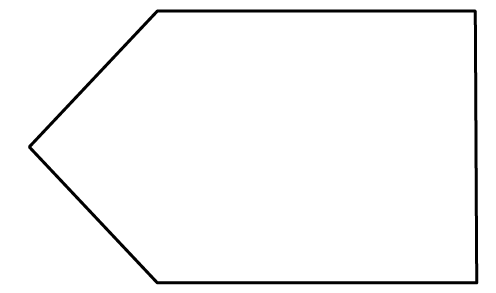(vii)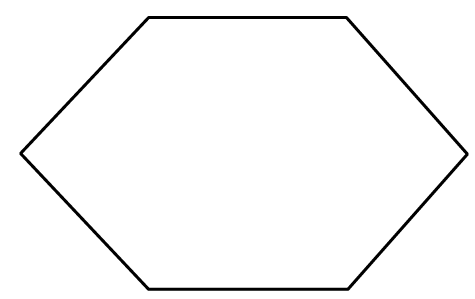(viii)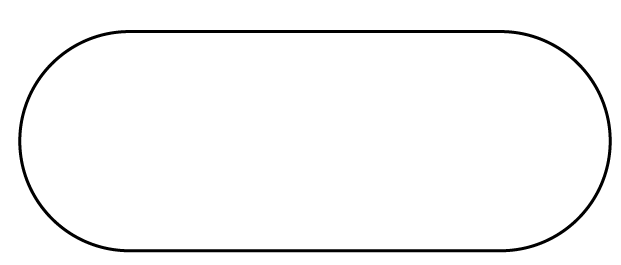Solution:

A plane closed figure is defined as an adjoining figure made by joining three or more lines end to end

Here, in the above figures, the plane closed figures are (i), (iii), (iv), (v), (vi), (vii) and (viii).

2. Fill in the blanks:

(i) …… is a three sided plane closed figure

(ii) A square is a plane closed figure which is not bounded by ……….

(iii) A rectangle is a ……. sided plane ……..

(iv) A rectangle has opposite sides ….. and adjacent sides ……… to each other.

(v) The sides of a square are …… to each other and each angle is ……….

Solution:

(i) Triangle is a three sided plane closed figure

(ii) A square is a plane closed figure which is not bounded by any curved line

(iii) A rectangle is a four sided plane closed figure

(iv) A rectangle has opposite sides equal and parallel and adjacent sides unequal and perpendicular to each other

(v) The sides of a square are equal to each other and each angle is 900

3. State, which of the lines/line – segments are perpendicular to the line PQ: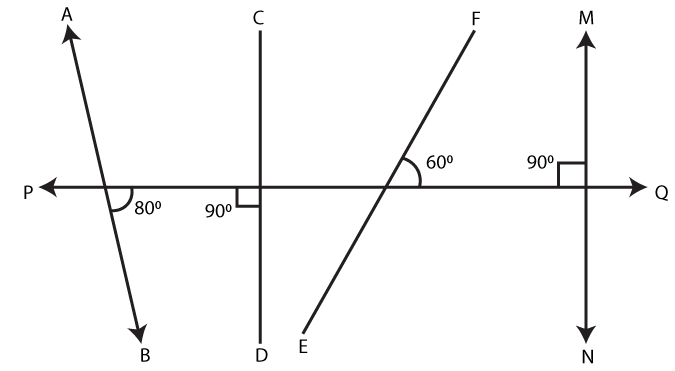Solution:

Two lines are said to be perpendicular when they meet or intersect each other at right angles

Hence, in the above figure, CD and MN are the lines which are perpendicular to the line PQ, forming an angle 900

4. Which of the following figures show two mutually perpendicular lines:

(i)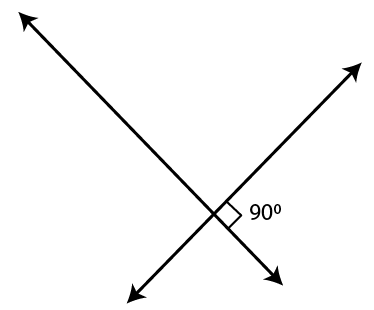(ii)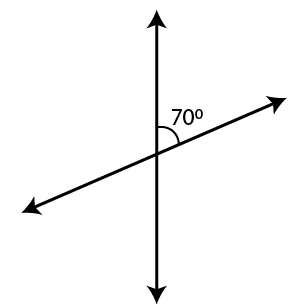(iii)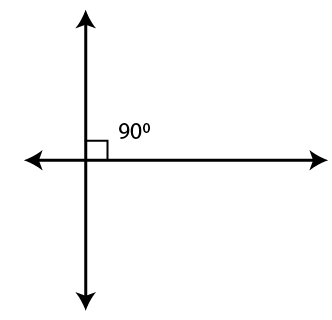Solution:

When both the lines are intersecting each other at right angles, then the lines are said to be mutually perpendicular lines.

Figures (i) and (iii) are mutually perpendicular lines forming a right angle

5. For each figure given below name the line segment that is perpendicular bisector of the other:

(i)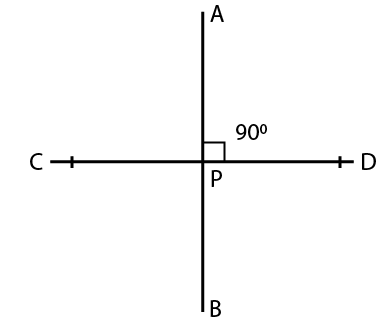(ii)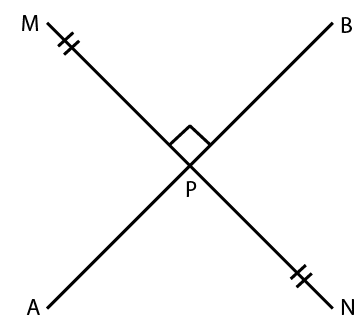(iii)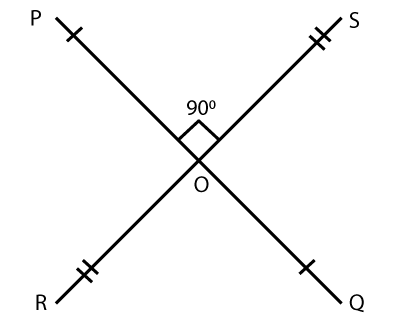(iv)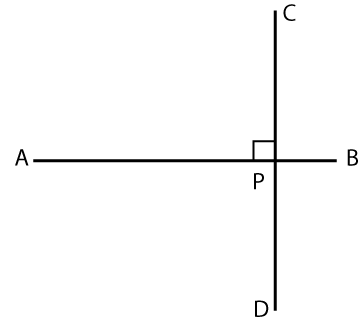(v)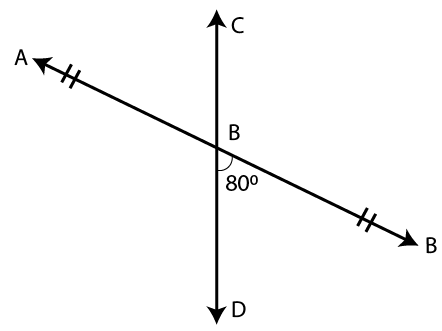Solution:

Perpendicular bisector is a line that divides a line segment into two equal parts and makes a right angle

(i) AB is the perpendicular bisector of CD

(ii) AB is the perpendicular bisector of MN

(iii) PQ and RS are both perpendicular bisector to each other

(iv) No line is perpendicular bisector to the other

(v) No line is perpendicular bisector to the other

6. Name three objects from your surroundings that contain perpendicular edges.

Solution:

Black board, scale and note-book contain perpendicular edges

7. Using the given figure, answer the following:

(i) Name the pairs of parallel lines

(ii) Name the pairs of mutually perpendicular lines

(iii) Is the line p parallel to the line l?

(iv) Is the line q perpendicular to the line m?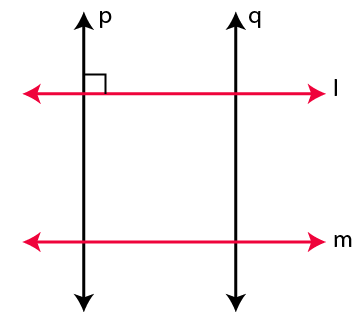Solution:

(i) Parallel lines are the lines which lie in the same plane but never intersect each other

l || m and p || q are the pairs of parallel lines in the above figure

(ii) Mutually perpendicular lines are the lines which intersect each other forming a right angle.

P and l, p and m, q and l, q and m are mutually perpendicular lines in the above figure

(iii) No, p is perpendicular to the line l

(iv) Yes, q is perpendicular to the line m

8. Place a scale (ruler) on a sheet of paper and hold it firmly with one hand. Now draw two line segments AB and CD along the longer edges of the scale. State whether segment AB is parallel to or perpendicular to segment CD.

Solution: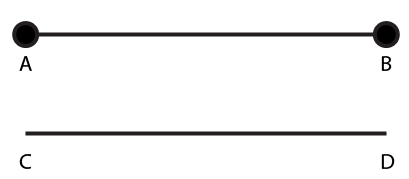As per the given statement AB || CD as shown in the figure

(i) How many pairs of its edges are parallel to each other?

(ii) How many pairs of its edges are perpendicular to each other?

Solution:

(i) 8 edges are parallel to each other

(ii) 8 edges are parallel to each other and 3 edges perpendicular to each other

= 8 × 3

= 24 edges

10. Give two examples from your surroundings for each of the following:

(i) intersecting lines

(ii) parallel lines

(iii) perpendicular lines.

Solution:

(i) The edges of textbook and notebook through any corner are intersecting lines

(ii) Opposite edges of textbook and notebook through any corner are parallel lines

(iii) The adjacent edges of textbook and black board in a class room through any corner are perpendicular lines

11. State true or false, if false, give the correct statement:

(i) The maximum number of lines through three collinear points is three.

(ii) The maximum number of lines through three non-collinear points is three.

(iii) Two parallel lines always lie in the same plane.

(iv) Concurrent lines always meet at the same point.

(v) A surface can be plane or curved.

Solution:

(i) False. As only one line can pass through three collinear points

(ii) False. It is not possible to pass any line through three non collinear points

(iii) True

(iv) True

(v) True